### NCERT Solutions For Class 11 Math Chapter -2 Exercise – 2.3

NCERT Solutions For Class 11 Math Chapter – 2 Exercise – 2.3

1. Which of the following relations are functions? Give reasons. If it is a function, determine its domain and range.

(i) {(2, 1), (5, 1), (8, 1), (11, 1), (14, 1), (17, 1)}

(ii) {(2, 1), (4, 2), (6, 3), (8, 4), (10, 5), (12, 6), (14, 7)}

(iii) {(1, 3), (1, 5), (2, 5)}

Solution:

(i) {(2, 1), (5, 1), (8, 1), (11, 1), (14, 1), (17, 1)}

As 2, 5, 8, 11, 14, and 17 are the elements of the domain of the given relation having their unique images, this relation can be called as a function.

Here,

domain = {2, 5, 8, 11, 14, 17} and range = {1}

(ii) {(2, 1), (4, 2), (6, 3), (8, 4), (10, 5), (12, 6), (14, 7)}

As 2, 4, 6, 8, 10, 12, and 14 are the elements of the domain of the given relation having their unique images, this relation can be called as a function.

Here, domain = {2, 4, 6, 8, 10, 12, 14} and range = {1, 2, 3, 4, 5, 6, 7}

(iii) {(1, 3), (1, 5), (2, 5)}

It’s seen that the same first element i.e., 1 corresponds to two different images i.e., 3 and 5, this relation cannot be called as a function.

2. Find the domain and range of the following real function:

(i) f(x) = –|x| (ii) f(x) = √(9 – x2

Solution:

(i) Given,

f(x) = –|x|, x ∈ R

We know that,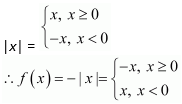As f(x) is defined for x ∈ R, the domain of f is R.

It is also seen that the range of f(x) = –|x| is all real numbers except positive real numbers.

Therefore, the range of f is given by (–∞, 0].

(ii) f(x) = √(9 – x2)

As √(9 – x2) is defined for all real numbers that are greater than or equal to –3 and less than or equal to 3, for 9 – x2 ≥ 0.

So, the domain of f(x) is {x: –3 ≤ x ≤ 3} or [–3, 3].

Now,

For any value of x in the range [–3, 3], the value of f(x) will lie between 0 and 3.

Therefore, the range of f(x) is {x: 0 ≤ x ≤ 3} or [0, 3].

3. A function f is defined by f(x) = 2x – 5. Write down the values of

(i) f(0), (ii) f(7), (iii) f(–3)

Solution:

Given,

Function, f(x) = 2x – 5.

Therefore,

(i) f(0) = 2 × 0 – 5 = 0 – 5 = –5

(ii) f(7) = 2 × 7 – 5 = 14 – 5 = 9

(iii) f(–3) = 2 × (–3) – 5 = – 6 – 5 = –11

4. The function ‘t’ which maps temperature in degree Celsius into temperature in degree Fahrenheit is defined by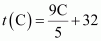.

Find (i) t (0) (ii) t (28) (iii) t (–10) (iv) The value of C, when t(C) = 212

Solution: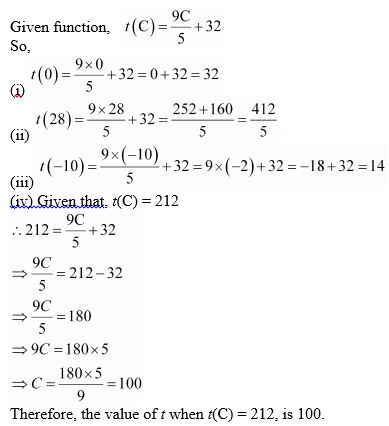5. Find the range of each of the following functions.

(i) f(x) = 2 – 3xx ∈ R, x > 0.

(ii) f(x) = x2 + 2, x is a real number.

(iii) f(x) = xx is a real number.

Solution:

(i) Given,

f(x) = 2 – 3xx ∈ R, x > 0.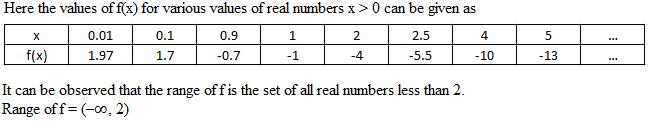We have,

x > 0

So,

3x > 0

-3x < 0 [Multiplying by -1 both the sides, the inequality sign changes]

2 – 3x < 2

Therefore, the value of 2 – 3x is less than 2.

Hence, Range = (–∞, 2)

(ii) Given,

f(x) = x2 + 2, x is a real number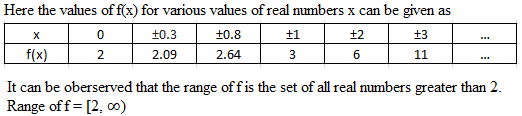We know that,

x2 ≥ 0

So,

x2 + 2 ≥ 2 [Adding 2 both the sides]

Therefore, the value of x2 + 2 is always greater or equal to 2 for x is a real number.

Hence, Range = [2, ∞)

(iii) Given,

f(x) = x, x is a real number

Clearly, the range of f is the set of all real numbers.

Thus,

Range of f = R